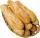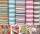# Infinite decimal

Imagine the infinite decimal number 0.99999999 .. ... ... ... That is a decimal and her endless serie of nines. Determine how much this number is less than the number 1. Thank you in advance.

Result

x =  0

#### Solution:Leave us a comment of example and its solution (i.e. if it is still somewhat unclear...):

Showing 0 comments:Be the first to comment!#### To solve this example are needed these knowledge from mathematics:

Need help calculate sum, simplify or multiply fractions? Try our fraction calculator.

## Next similar examples:

1. Decimal to fractionWrite decimal number 8.638333333 as a fraction A/B in the basic form. Given decimal has infinite repeating figures.
2. Series and sequencesFind a fraction equivalent to the recurring decimal? 0.435643564356
3. FractionFraction ? write as fraction a/b, a, b is integers numerator/denominator.
4. Sum of seriesDetermine the 6-th member and the sum of a geometric series: 5-4/1+16/5-64/25+256/125-1024/625+....
5. Theorem proveWe want to prove the sentense: If the natural number n is divisible by six, then n is divisible by three. From what assumption we started?
6. Reduce to lowest termsReduce to lowest terms : 32/124
7. RollsMom bought 13 rolls. Dad ate 3.5 rolls. How many rolls left when Peter yet put two at dinner?
8. PackageThe package was 23 meters of textile. The first day sold 12.3 meters. How many meters of textile remained in the package?
9. Geometric progression 2There is geometric sequence with a1=5.7 and quotient q=-2.5. Calculate a17.
10. Six termsFind the first six terms of the sequence a1 = -3, an = 2 * an-1
11. Five membersWrite first 5 members geometric sequence and determine whether it is increasing or decreasing: a1 = 3 q = -2
12. A perineumA perineum string is 10% shorter than its original string. The first string is 24, what is the 9th string or term?
13. Geometric progression 48,4√2,4,2√2
14. GP membersThe geometric sequence has 10 members. The last two members are 2 and -1. Which member is -1/16?
15. GP - 8 itemsDetermine the first eight members of a geometric progression if a9=512, q=2
16. Geometric sequence 4It is given geometric sequence a3 = 7 and a12 = 3. Calculate s23 (= sum of the first 23 members of the sequence).
17. Tenth memberCalculate the tenth member of geometric sequence when given: a1=1/2 and q=2